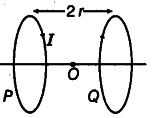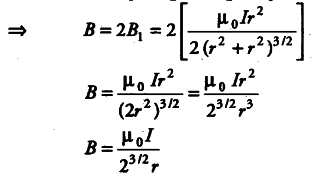# Two identical circular loops P and Q

Two identical circular loops P and Q, each of radius r and carrying equal currents are kept in the parallel planes having a common axis passing through O. The direction of current in P is clockwise and in Q is anti-clockwise as seen from O, which is equidistant from the loops P and Q. Find the magnitude of the net magnetic field at O.Magnetic field at O due to two loops will be in same direction (Q—> P, along the axis) and of equal magnitude.
B = { B }_{ 1 } +
{ B }_{ 2 } or
{ B }_{ 1 } =
{ B }_{ 2 }